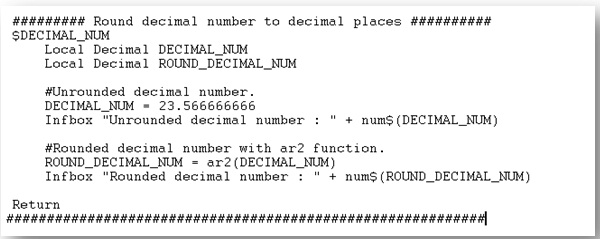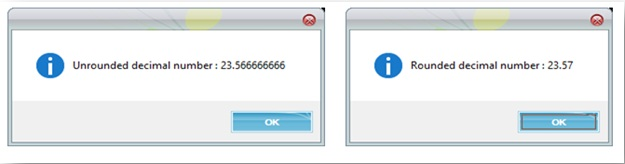# How to roundup decimal number to two decimal places

By | September 23, 2014

In a situation dealing with arithmetic operations, you may want to roundup the calculation result to up to two decimal places. Sage X3 has a special function to roundup a decimal number to two decimal places.

ar2: This function roundups a decimal number to two decimal places.

Syntax:  ar2(exp_num)

Parameter details: exp_num: Decimal number that will pass to “ar2” function. Function will return rounded two decimal digit number. Kindly refer to the below snippet of code for better understanding.